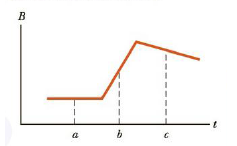Chapter 20.2, Problem 20.1QQ

Chapter
Section
Textbook Problem

Figure 20.6 is a graph of the magnitude B versus time for a magnetic field that passes through a fixed loop and is oriented perpendicular to the plane of the loop. Rank the magnitudes of the emf generated in the loop from largest to smallest at the three instants indicated.Figure 20.6 (Quick Quiz 20.1)

To determine
The ranking of the points based on the emf generated.

Explanation

The emf generated is given by

ε=AΔBΔt

• A is the area of the loop.
• B is the magnetic field.

The slope of the graph gives the rate of change of magnetic field. The magnitude of emf is proportional to the absolute value of the slope.

At the point a, the magnetic field is constant

Still sussing out bartleby?

Check out a sample textbook solution.

See a sample solution

The Solution to Your Study Problems

Bartleby provides explanations to thousands of textbook problems written by our experts, many with advanced degrees!

Get Started